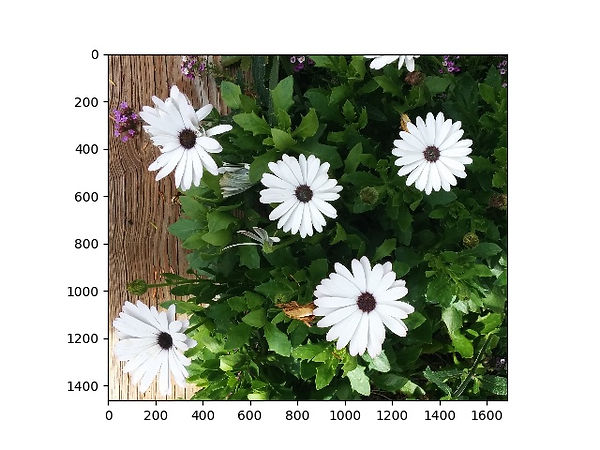top of page

# Summary

### Image Files

The picture of flowers to the right is what you’re used to thinking of as an image. But to a computer, an image is a series of numbers that are organized in a specific way. We demonstrate this in the code block below:

• "Flowers.jpg" is the name of the image. If you want to run the code, you can right-click and save the image to the right.

• To turn this image into a form that Python can read, we will use the free and publicly available scikit-image library (also sometimes called a package). Lesson 2 taught us that a library is a collection of code that other people can use as part of their own software projects. Note that scikit-image is imported as “skimage” because the name used inside Python is not necessarily the same as the name that we call a package in writing.• Within the scikit-image library is a function called io.imread. When you pass your filename (which is a string) to the io.imread function, the function will return a NumPy array (notice that the scikit-image library itself uses the NumPy library). For a refresher on NumPy arrays, see Lesson 2. In order to do more with the NumPy array holding the image information, we need to store it in the variable that we choose to call “imagefile.” (Note that running the code will be a little slow because the scikit-image package has to be downloaded by replit; however, if you're working with a Python installation on your own computer, you only have to download and install new packages the first time you use them).

• Since “imagefile” is a NumPy array, we can print out its shape, which is (1464, 1687, 3). 1464 represents the number of pixels in the image in the vertical direction. 1687 represents the number of pixels in the horizontal direction. Finally, 3 represents the number of colors that we can break this image down into: red, green, and blue (in that order). It is very common for computers to represent colors as some combination of these three colors. This is known as RGB for short. Each of the three values should be an integer from 0 to 255. The larger the number, the more the individual color contributes to the overall color. If all three values are 0, the pixel is black. If they are all 255, the pixel is white.#import image processing package

from skimage import io

print("The datatype of the image is", type(imagefile))

print("The shape of the image array is", imagefile.shape)

We can see what the array looks like with the statement “print(imagefile).” Feel free to try it above! It will print with ellipses because the array is so large, so this isn’t a good strategy for looking up what values correspond to what pixels in the image.

However, since an image in Python is just a NumPy array, we can use the same functions that we would for other NumPy arrays. For example, we can select part of the image by using normal indexing. We can figure out the color of the pixel at a vertical position of 1200 and a horizontal position of 1491with the command “imagefile[1200,1491].”

Can you determine the color of the pixel at (1200,1000)?

bottom of page